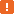# Integer (%INT) Macro#### Description

The Integer macro is used to insert integer numbers into strings generated with the Custom String generator.

The macro is an analog of the Integer data generator.

#### Syntax

The macro has the following syntax:

%INT(MinValue, MaxValue, Increment, *SEQ|*RND, *xN)

#### Parameters

MinValue and MaxValue

Optional parameters. Specify the lower and upper bounds of integer numbers to be generated.

 Note: The value of the MaxValue parameter must be greater than or equal to the value of the MinValue parameter. Default values: MinValue = 1 and MaxValue = 2147483647.

Increment

Specifies an increment value for the generated sequence. Note that if you want to generate a descending sequence, you need to specify a negative value for this parameter. The default value of the parameter is 1.This parameter is used only with the *Sequential generation mode.

*SEQ or *RND

Optional parameter. Specifies the generation mode:

• *SEQ (or *Sequential) - Default. If this mode is used, TestComplete generates a sequence of values that will start from the value of the MinValue parameter. The increment value of the sequence is specified by the Increment parameter.
• *RND (or *Random) - If this mode is used, TestComplete generates random integer number values within the specified range.

*xN

Optional parameter. Specifies the number of possible repetitions of each value in series of generated strings. N is a positive number that specifies the number of repetitions: 1, 2, 3, and so on. The default parameter value is `*x1` (no repetitions).

#### Examples

The following example demonstrates how to use the Integer macro:

• %INT(1, 10000, 20)

Generates a sequence of values starting from 1 with the increment value of 20.

• %INT(1, 10000, -50)

Generates a sequence of values starting from 10000 with the increment value of -50.

• %INT(1, 10000, 15, *RND)

Generates a random collection of values from 1 to 10000. In this case, the value of the Increment parameter (15) is omitted.

• user%.3d%INT(1,100,1)

Uses the `%.3d` format specifier to generate the following sequence of values: user001, user002, user003 and so on.

• %INT(1, 10000, -3, *x2)

Generates a sequence of values starting from 10000 with the increment value of -3. Each of the generated values will be repeated twice.

• %INT()

Generates a sequence of values starting from 1 with the increment value of 1 (default parameter values).

• %INT(*Random, *x3)

Generates a random collection of values from 1 to 2147483647. Each generated value will be repeated three times.

#### Remarks

• If some numeric parameters are specified, the macro requires that the first numeric parameter be the value of the MinValue parameter. The second numeric parameter must be the value of the MaxValue parameter. If you specify one more numeric parameter, it will be interpreted as the value of the Increment parameter. In this case, the *Sequential generation mode will be used automatically.